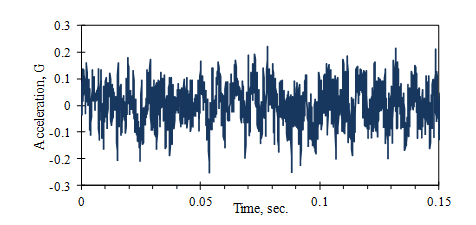# Statistics & Probabilities Intro

March 29, 2018

A random vibration signal seems hard to describe quantitatively. Just answering the question, “How big is it?” is challenging. This sections covers some of the ways random signals are quantified, including statistical measures (such as the mean value) and probability distributions (such as the Normal curve). The course also covers product design applications, such as  tolerances and fatigue analysis.Figure 1: Random Vibration Signal (Acceleration of Car Floor at 120 km/h)

In this section it is assumed that a continuous vibration signal, x(t), has been digitized into a sequence of numbers, xnn = 1, …, N,  used for the analysis.